# 十个经典排序算法（时间复杂度，空间复杂度，稳定性，动画演示思想）

2019/03/25 21:03

 类型 时间复杂度 空间复杂度 冒泡 O(n^2) O(1) 选择 O(n^2) O(1) 插入 O(n^2) O(1) 归并 O(n*logn) O(N) 快速 O(n*logn) O(logN)~O(N) 堆 O(n*logn) O(1) 希尔 O(n*logn) O(1)

 类型 时间复杂度 空间复杂度 计数排序 O(N) O(N) 基数排序 O(N) O(N) 桶排序 O(N) O(N)

假定待排序的记录序列中，存在多个具有相同的关键字的记录，若经过排序，这些记录的相对次序保存不变，称这种排序算法是稳定的，否则称为不稳定的。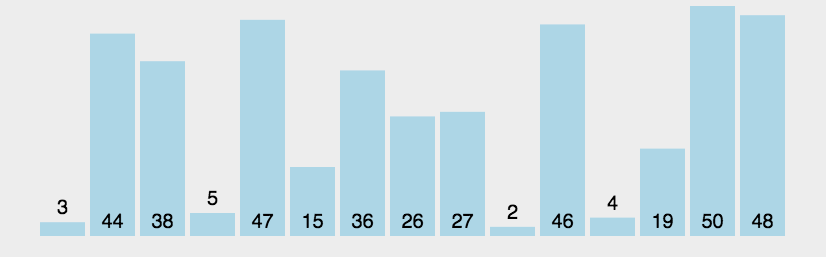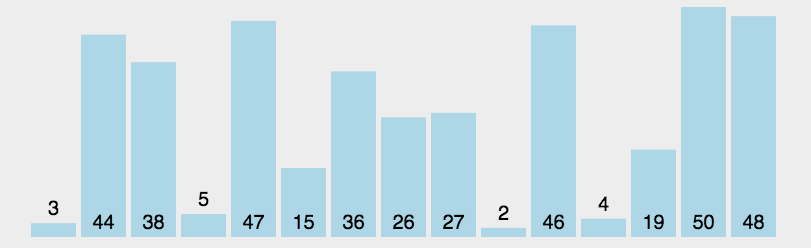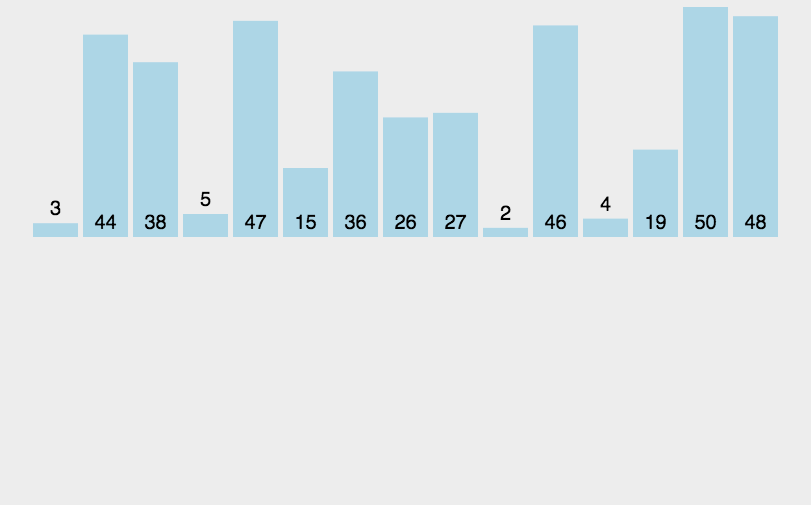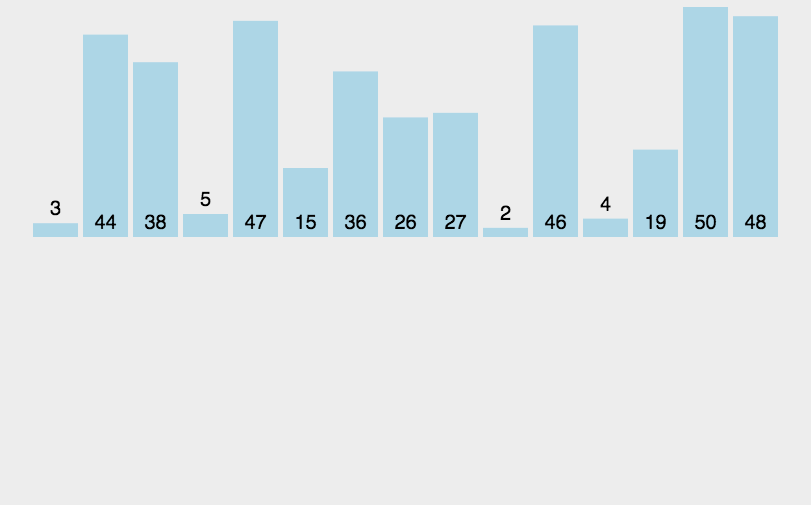• 默认选择第一个数为基数
• 实现比基数小的数放在基数左边，比基数大的数放在基数右边
• 设置左右两个指标，不断向中间靠，左边寻找比基数大的数，标记，右边寻找比基数小的数，标记，交换两个标记位置的数
• 直到两个指标相遇，停止移动指标，交换基数位和左指标位置的数
• 继续重复以上步骤，对两个区别的数进行同等操作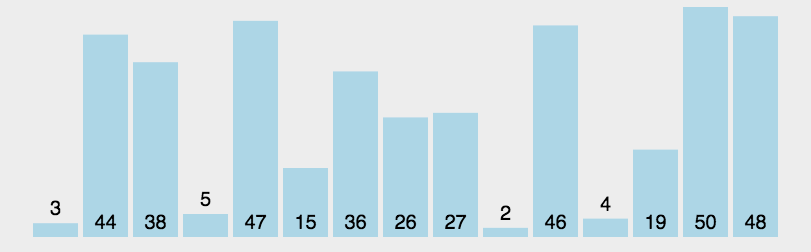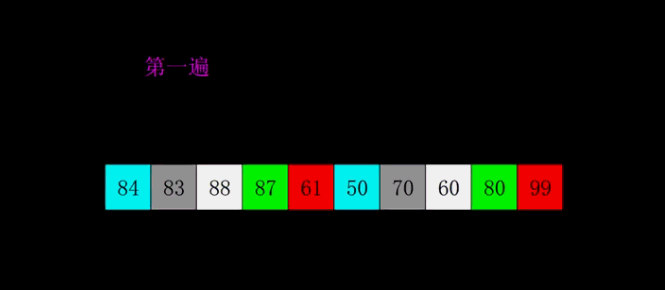• 建成大根堆
• 堆顶元素和最后一个元素交换
• 剔除最后一个元素
• 变成大根堆，重复2,3步骤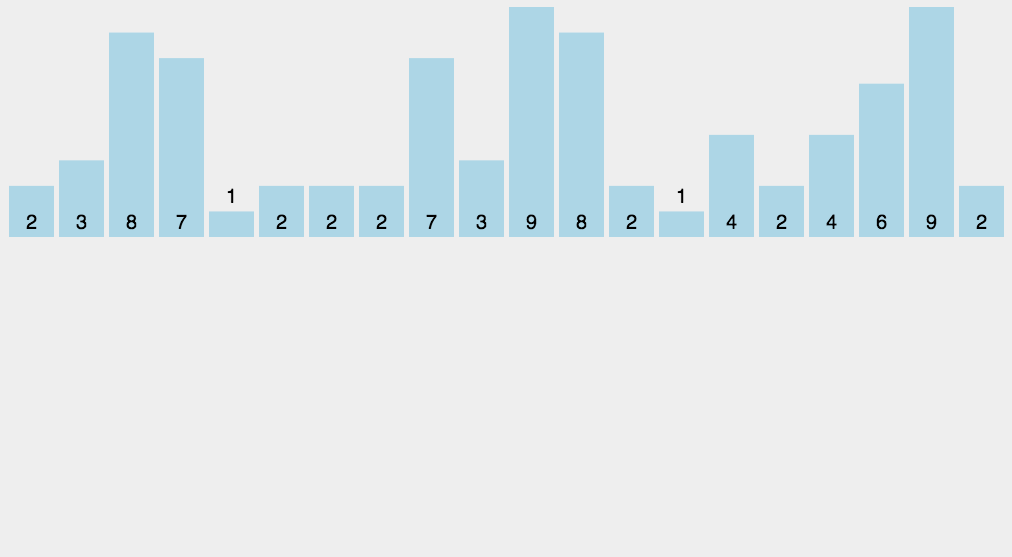• 设置一个定量的数组当作空桶；
• 遍历输入数据，并且把数据一个一个放到对应的桶里去；
• 对每个不是空的桶进行排序；
• 从不是空的桶里把排好序的数据拼接起来。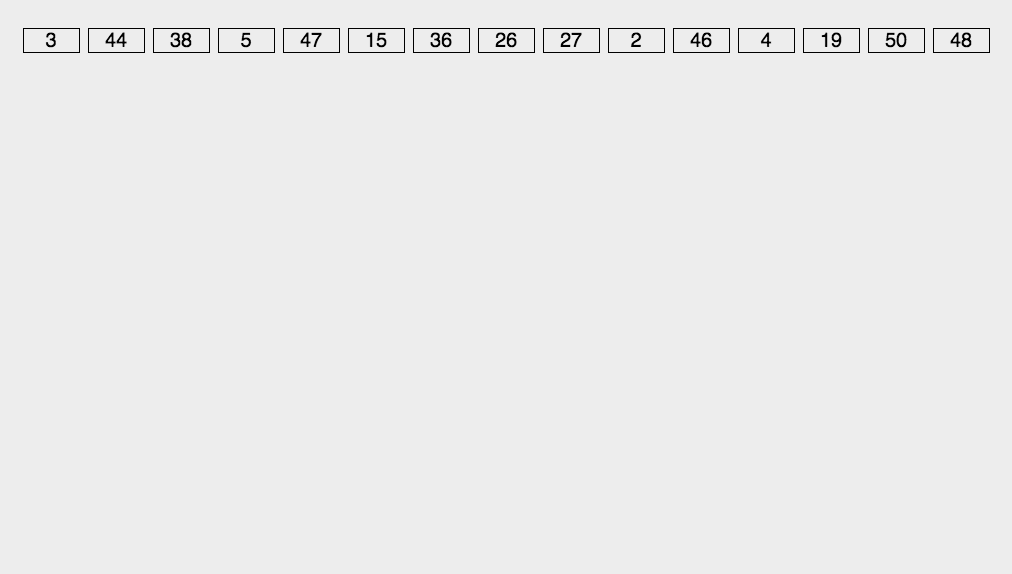0
0 收藏

### 作者的其它热门文章0 评论
0 收藏
0